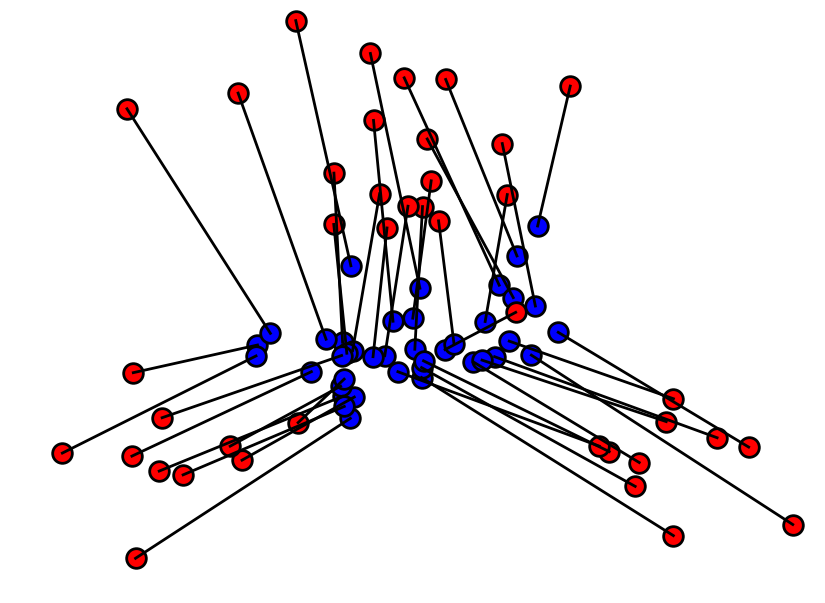# Optimal Transport with Linear Programming¶

Important: Please read the installation page for details about how to install the toolboxes. $\newcommand{\dotp}{\langle #1, #2 \rangle}$ $\newcommand{\enscond}{\lbrace #1, #2 \rbrace}$ $\newcommand{\pd}{ \frac{ \partial #1}{\partial #2} }$ $\newcommand{\umin}{\underset{#1}{\min}\;}$ $\newcommand{\umax}{\underset{#1}{\max}\;}$ $\newcommand{\umin}{\underset{#1}{\min}\;}$ $\newcommand{\uargmin}{\underset{#1}{argmin}\;}$ $\newcommand{\norm}{\|#1\|}$ $\newcommand{\abs}{\left|#1\right|}$ $\newcommand{\choice}{ \left\{ \begin{array}{l} #1 \end{array} \right. }$ $\newcommand{\pa}{\left(#1\right)}$ $\newcommand{\diag}{{diag}\left( #1 \right)}$ $\newcommand{\qandq}{\quad\text{and}\quad}$ $\newcommand{\qwhereq}{\quad\text{where}\quad}$ $\newcommand{\qifq}{ \quad \text{if} \quad }$ $\newcommand{\qarrq}{ \quad \Longrightarrow \quad }$ $\newcommand{\ZZ}{\mathbb{Z}}$ $\newcommand{\CC}{\mathbb{C}}$ $\newcommand{\RR}{\mathbb{R}}$ $\newcommand{\EE}{\mathbb{E}}$ $\newcommand{\Zz}{\mathcal{Z}}$ $\newcommand{\Ww}{\mathcal{W}}$ $\newcommand{\Vv}{\mathcal{V}}$ $\newcommand{\Nn}{\mathcal{N}}$ $\newcommand{\NN}{\mathcal{N}}$ $\newcommand{\Hh}{\mathcal{H}}$ $\newcommand{\Bb}{\mathcal{B}}$ $\newcommand{\Ee}{\mathcal{E}}$ $\newcommand{\Cc}{\mathcal{C}}$ $\newcommand{\Gg}{\mathcal{G}}$ $\newcommand{\Ss}{\mathcal{S}}$ $\newcommand{\Pp}{\mathcal{P}}$ $\newcommand{\Ff}{\mathcal{F}}$ $\newcommand{\Xx}{\mathcal{X}}$ $\newcommand{\Mm}{\mathcal{M}}$ $\newcommand{\Ii}{\mathcal{I}}$ $\newcommand{\Dd}{\mathcal{D}}$ $\newcommand{\Ll}{\mathcal{L}}$ $\newcommand{\Tt}{\mathcal{T}}$ $\newcommand{\si}{\sigma}$ $\newcommand{\al}{\alpha}$ $\newcommand{\la}{\lambda}$ $\newcommand{\ga}{\gamma}$ $\newcommand{\Ga}{\Gamma}$ $\newcommand{\La}{\Lambda}$ $\newcommand{\si}{\sigma}$ $\newcommand{\Si}{\Sigma}$ $\newcommand{\be}{\beta}$ $\newcommand{\de}{\delta}$ $\newcommand{\De}{\Delta}$ $\newcommand{\phi}{\varphi}$ $\newcommand{\th}{\theta}$ $\newcommand{\om}{\omega}$ $\newcommand{\Om}{\Omega}$

This numerical tours details how to solve the discrete optimal transport problem (in the case of measures that are sums of Diracs) using linear programming.

In :
using PyPlot
using NtToolBox


## Optimal Transport of Discrete Distribution¶

We consider two dicretes distributions $$\forall k=0,1, \quad \mu_k = \sum_{i=1}^{n_k} p_{k,i} \de_{x_{k,i}}$$ where $n_0,n_1$ are the number of points, $\de_x$ is the Dirac at location $x \in \RR^d$, and $X_k = ( x_{k,i} )_{i=1}^{n_k} \subset \RR^d$ for $k=0,1$ are two point clouds.

We define the set of couplings between $\mu_0,\mu_1$ as

$$\Pp = \enscond{ (\ga_{i,j})_{i,j} \in (\RR^+)^{n_0 \times n_1} }{ \forall i, \sum_j \ga_{i,j} = p_{0,i}, \: \forall j, \sum_i \ga_{i,j} = p_{1,j} }$$

The Kantorovitch formulation of the optimal transport reads

$$\ga^\star \in \uargmin{\ga \in \Pp} \sum_{i,j} \ga_{i,j} C_{i,j}$$

where $C_{i,j} \geq 0$ is the cost of moving some mass from $x_{0,i}$ to $x_{1,j}$.

The optimal coupling $\ga^\star$ can be shown to be a sparse matrix with less than $n_0+n_1-1$ non zero entries. An entry $\ga_{i,j}^\star \neq 0$ should be understood as a link between $x_{0,i}$ and $x_{1,j}$ where an amount of mass equal to $\ga_{i,j}^\star$ is transfered.

In the following, we concentrate on the $L^2$ Wasserstein distance. $$C_{i,j}=\norm{x_{0,i}-x_{1,j}}^2.$$

The $L^2$ Wasserstein distance is then defined as $$W_2(\mu_0,\mu_1)^2 = \sum_{i,j} \ga_{i,j}^\star C_{i,j}.$$

The coupling constraint $$\forall i, \sum_j \ga_{i,j} = p_{0,i}, \: \forall j, \sum_i \ga_{i,j} = p_{1,j}$$ can be expressed in matrix form as $$\Sigma(n_0,n_1) \ga = [p_0;p_1]$$ where $\Sigma(n_0,n_1) \in \RR^{ (n_0+n_1) \times (n_0 n_1) }$.

In :
Cols  = (n0,n1) -> sparse(vec(repeat(1:n1, outer=(1,n0))'), vec(reshape(collect(1:n0*n1),n0,n1) ), Int.(ones(n0*n1)) );

Rows  = (n0,n1) -> sparse( vec(repeat(1:n0, outer=(1,n1))'), vec(reshape(collect(1:n0*n1),n0,n1)' ), Int.(ones(n0*n1) ));
Sigma = (n0,n1) -> [Rows(n0,n1); Cols(n0,n1)];


We use a simplex algorithm to compute the optimal transport coupling $\ga^\star$.

In :
maxit = 1e4
tol = 1e-9
otransp = (C,p0,p1) -> reshape(perform_linprog(Array(Sigma(length(p0),length(p1))),[vec(p0);vec(p1)], C, maxit, tol), length(p0), length(p1));


Dimensions $n_0, n_1$ of the clouds.

In :
n0 = 60
n1 = 80;


Compute a first point cloud $X_0$ that is Gaussian, and a second point cloud $X_1$ that is Gaussian mixture.

In :
gauss = (q,a,c) -> a*randn(2, q) + repeat(c', outer=(1,q))
X0 = randn(2,n0)*.3
X1 = [gauss(Base.div(n1,2),.5, [0 1.6]) gauss(Base.div(n1,4),.3, [-1 -1]) gauss(Base.div(n1,4),.3, [1 -1])];


Density weights $p_0, p_1$.

In :
normalize = a-> a/sum(a)
p0 = normalize(rand(n0, 1))
p1 = normalize(rand(n1, 1));


Shortcut for display.

In :
myplot = (x,y,ms,col) -> scatter(x,y, s=ms*20, edgecolors="k", c=col, linewidths=2);


Display the point clouds. The size of each dot is proportional to its probability density weight.

In :
figure(figsize = (10,7))
axis("off")

for i in 1:length(p0)
myplot(X0[1,i], X0[2,i], p0[i]*length(p0)*10, "b")
end

for i in 1:length(p1)
myplot(X1[1,i], X1[2,i], p1[i]*length(p1)*10, "r")

xlim(minimum(X1[1,:])-.1,maximum(X1[1,:])+.1)
ylim(minimum(X1[2,:])-.1,maximum(X1[2,:])+.1)
end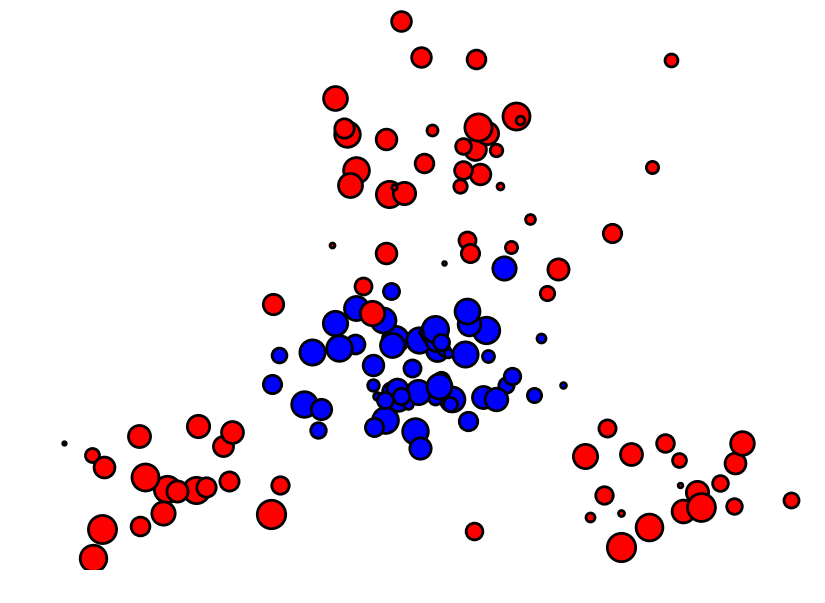Compute the weight matrix $(C_{i,j})_{i,j}.$

In :
C = repeat( sum(X0.^2,1)', outer=(1, n1) ) + repeat( sum(X1.^2,1), outer=(n0,1) ) - 2*X0'*X1;

In :
Gamma = otransp(C, p0, p1);


Check that the number of non-zero entries in $\ga^\star$ is $n_0+n_1-1$.

In :
println("Number of non-zero: $(length(Gamma[Gamma.>0])) (n0 + n1-1 =$(n0 + n1-1))" )

Number of non-zero: 139 (n0 + n1-1 = 139)


Check that the solution satifies the constraints $\ga \in \Cc$.

In :
println("Constraints deviation (should be 0): $(norm(sum(Gamma,2)-vec(p0))),$(norm(sum(Gamma, 1)'-vec(p1)))")

Constraints deviation (should be 0): 4.9279582884301896e-17, 7.359808400080195e-18


## Displacement Interpolation¶

For any $t \in [0,1]$, one can define a distribution $\mu_t$ such that $t \mapsto \mu_t$ defines a geodesic for the Wasserstein metric.

Since the $W_2$ distance is a geodesic distance, this geodesic path solves the following variational problem

$$\mu_t = \uargmin{\mu} (1-t)W_2(\mu_0,\mu)^2 + t W_2(\mu_1,\mu)^2.$$

This can be understood as a generalization of the usual Euclidean barycenter to barycenter of distribution. Indeed, in the case that $\mu_k = \de_{x_k}$, one has $\mu_t=\de_{x_t}$ where $x_t = (1-t)x_0+t x_1$.

Once the optimal coupling $\ga^\star$ has been computed, the interpolated distribution is obtained as

$$\mu_t = \sum_{i,j} \ga^\star_{i,j} \de_{(1-t)x_{0,i} + t x_{1,j}}.$$

Find the $i,j$ with non-zero $\ga_{i,j}^\star$.

In :
I,J,Gammaij = findnz(Gamma)

Out:
([32,13,45,56,26,10,32,8,18,25  …  53,1,52,35,55,31,60,1,41,58],[1,2,2,3,4,5,6,7,7,7  …  76,77,77,78,78,79,79,80,80,80],[0.0153754,0.00758918,0.00179225,0.00931836,0.00540233,0.000804079,0.00391476,0.00715802,0.00813156,0.000680036  …  0.0196654,0.0141461,0.00265769,0.00844133,0.00146386,0.00760516,0.00800825,0.0124461,0.00522099,0.00752341])

Display the evolution of $\mu_t$ for a varying value of $t \in [0,1]$.

In :
length(Gammaij)

Out:
139
In :
figure(figsize =(15,10))
tlist = collect(linspace(0, 1, 6))

for i in 1:length(tlist)
t = tlist[i]
Xt = (1-t)*X0[:,I] + t*X1[:,J]
subplot(2,3,i)
axis("off")
for j in 1:length(Gammaij)
myplot(Xt[1,j],Xt[2,j],Gammaij[j]*length(Gammaij)*6,[t,0,1-t])
end
title("t = $t") xlim(minimum(X1[1,:])-.1,maximum(X1[1,:])+.1) ylim(minimum(X1[2,:])-.1,maximum(X1[2,:])+.1) end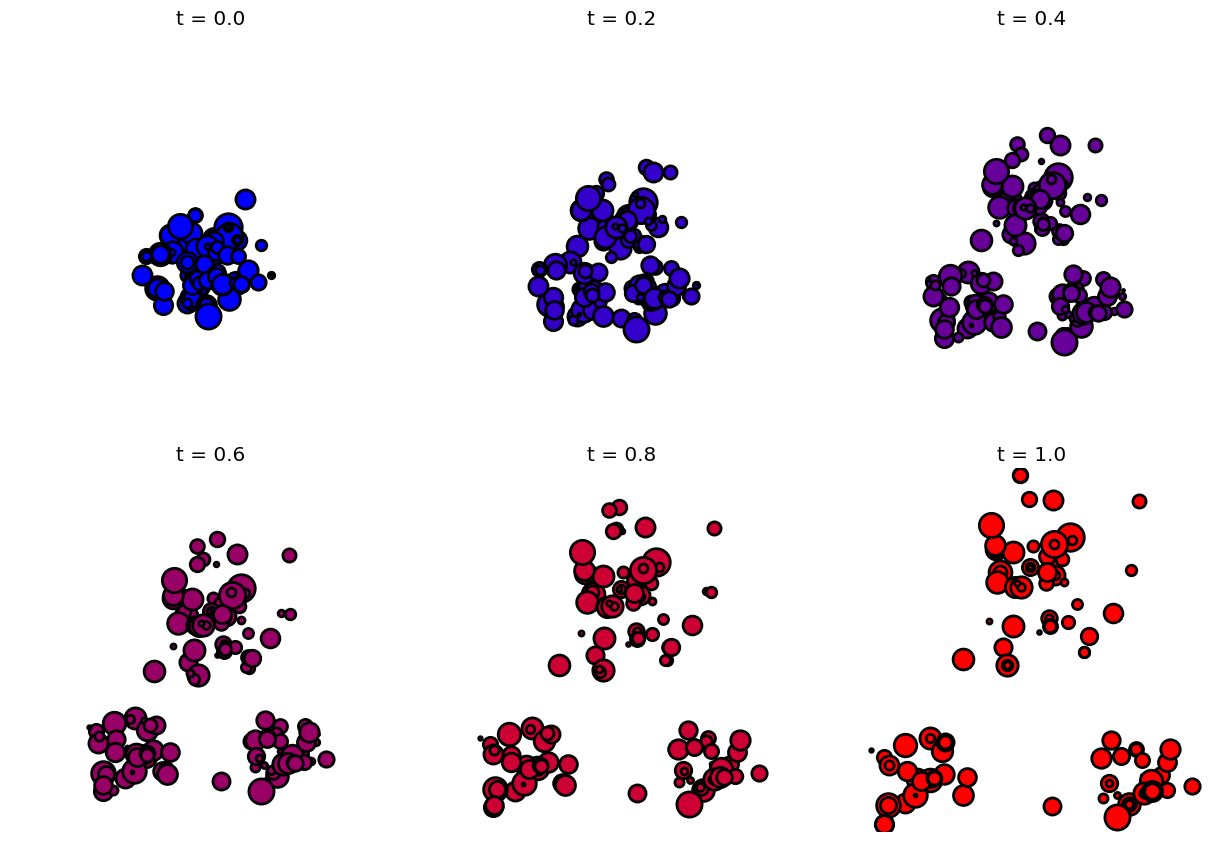## Optimal Assignement¶ In the case where the weights$p_{0,i}=1/n, p_{1,i}=1/n$(where$n_0=n_1=n$) are constants, one can show that the optimal transport coupling is actually a permutation matrix. This properties comes from the fact that the extremal point of the polytope$\Cc$are permutation matrices. This means that there exists an optimal permutation$ \si^\star \in \Sigma_n $such that $$\ga^\star_{i,j} = \choice{ 1 \qifq j=\si^\star(i), \\ 0 \quad\text{otherwise}. }$$ where$\Si_n$is the set of permutation (bijections) of$\{1,\ldots,n\}$. This permutation thus solves the so-called optimal assignement problem $$\si^\star \in \uargmin{\si \in \Sigma_n} \sum_{i} C_{i,\si(j)}.$$ Same number of points. In : n0 = 40 n1 = n0;  Compute points clouds. In : X0 = randn(2,n0)*.3 X1 = [gauss(Base.div(n1,2),.5, [0 1.6]) gauss(Base.div(n1,4),.3, [-1 -1]) gauss(Base.div(n1,4),.3, [1 -1])];  Constant distributions. In : p0 = ones(n0)/n0 p1 = ones(n1)/n1;  Compute the weight matrix$ (C_{i,j})_{i,j}. $In : C = repeat( sum(X0.^2,1)', outer=(1,n1) ) + repeat( sum(X1.^2,1), outer=(n0,1) ) - 2*X0'*X1;  Display the coulds. In : figure(figsize = (10,7)) axis("off") myplot(X0[1,:],X0[2,:],10,"b") myplot(X1[1,:],X1[2,:],10,"r") xlim(minimum(X1[1,:])-.1,maximum(X1[1,:])+.1) ylim(minimum(X1[2,:])-.1,maximum(X1[2,:])+.1);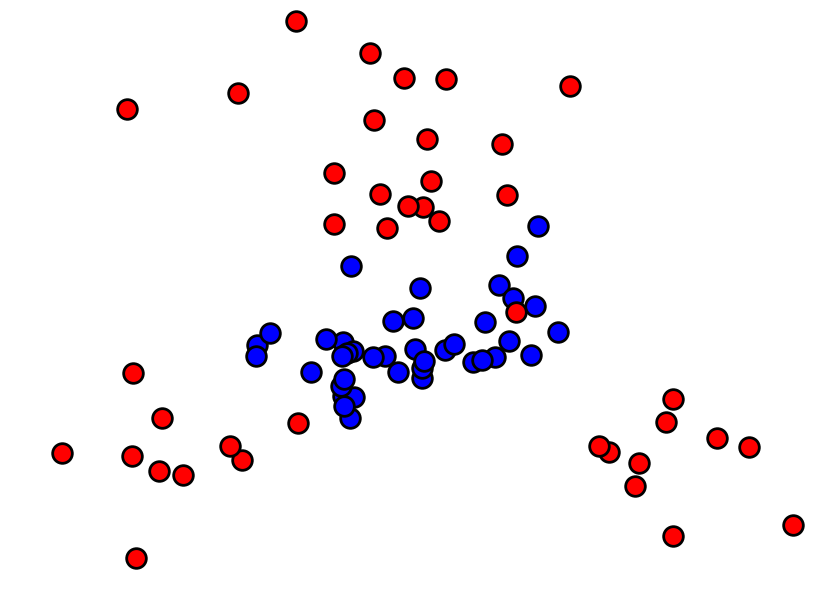Solve the optimal transport. In : Gamma = otransp(C, p0, p1);  Show that$\ga\$ is a binary permutation matrix.

In :
figure(figsize = (5,5))
imageplot(Gamma)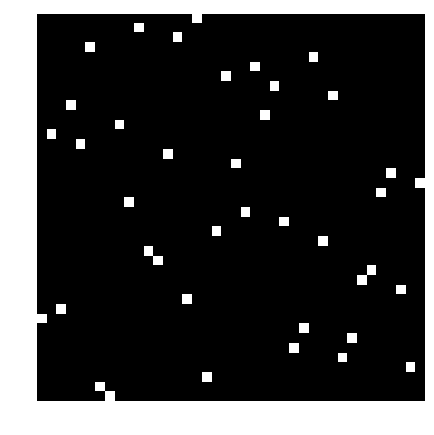Display the optimal assignement.

In :
I,J = findn(Gamma)

figure(figsize = (10,7))
axis("off")

for k in 1:length(I)
h = plot([X0[1,I[k]]; X1[1,J[k]]],[X0[2,I[k]]; X1[2,J[k]]],"k", lw = 2)
end

myplot(X0[1,:],X0[2,:],10,"b")
myplot(X1[1,:],X1[2,:],10,"r")

xlim(minimum(X1[1,:])-.1,maximum(X1[1,:])+.1)
ylim(minimum(X1[2,:])-.1,maximum(X1[2,:])+.1);